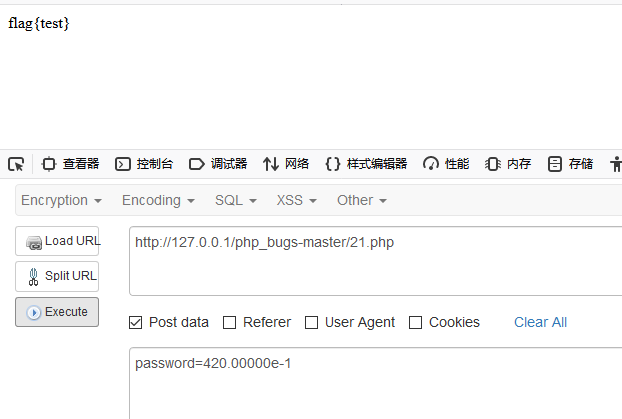zoukankan      html  css  js  c++  java
• # PHP代码审计分段讲解（8）

#### 20 十六进制与数字比较

源代码为：

```<?php

error_reporting(0);
function noother_says_correct(\$temp)
{
\$flag = 'flag{test}';
\$one = ord('1');  //ord — 返回字符的 ASCII 码值
\$nine = ord('9'); //ord — 返回字符的 ASCII 码值
\$number = '3735929054';
// Check all the input characters!
for (\$i = 0; \$i < strlen(\$number); \$i++)
{
// Disallow all the digits!
\$digit = ord(\$temp{\$i});
if ( (\$digit >= \$one) && (\$digit <= \$nine) )
{
// Aha, digit not allowed!
return "flase";
}
}
if(\$number == \$temp)
return \$flag;
}
echo noother_says_correct(\$temp);

?>```

我们很容易能够注意到：

`\$number == \$temp`

这里两个等号，是弱类型比较，即会先将比较的两者类型进行转化后再比较，在计算机中0x开头的表示为十六进制，所以我们可以使用十六进制来绕过前面的for循环，同时满足等于temp

#### 21 数字验证正则绕过

```<?php

error_reporting(0);
\$flag = 'flag{test}';
if  ("POST" == \$_SERVER['REQUEST_METHOD'])
{
if (0 >= preg_match('/^[[:graph:]]{12,}\$/', \$password)) //preg_match — 执行一个正则表达式匹配
{
echo 'Wrong Format';
exit;
}
while (TRUE)
{
\$reg = '/([[:punct:]]+|[[:digit:]]+|[[:upper:]]+|[[:lower:]]+)/';
if (6 > preg_match_all(\$reg, \$password, \$arr))
break;
\$c = 0;
\$ps = array('punct', 'digit', 'upper', 'lower'); //[[:punct:]] 任何标点符号 [[:digit:]] 任何数字  [[:upper:]] 任何大写字母  [[:lower:]] 任何小写字母
foreach (\$ps as \$pt)
{
\$c += 1;
}
if (\$c < 3) break;
//>=3，必须包含四种类型三种与三种以上
if ("42" == \$password) echo \$flag;
exit;
}
}

?>```

这里用到了PHP正则表达式，简单列举一下字符串类的正则表达式：

```[[:alpha:]] ：匹配任何字母
[[:digit:]] ：匹配任何数字
[[:alnum:]] ：匹配任何字母和数字
[[:space:]] ：匹配任何空白字符
[[:upper:]] ：匹配任何大写字母
[[:lower:]] ：匹配任何小写字母
[[:punct:]] ：匹配任何标点符号
[[:xdigit:]] ：匹配任何16进制数字，相当于[0-9a-fA-F]
[[:blank:]] ：匹配空格和Tab，等价于[	]
[[:cntrl:]] ：匹配所有ASCII 0到31之间的控制符
[[:graph:]] ：匹配所有的可打印字符，等价于[^

fv]
[[:print:]] ：匹配所有的可打印字符和空格，等价于[^

fv]```

```if  ("POST" == \$_SERVER['REQUEST_METHOD'])
{

第一个if中正则表达式匹配所有的可打印字符，并且需要大于等于12个

```    if (0 >= preg_match('/^[[:graph:]]{12,}\$/', \$password)) //preg_match — 执行一个正则表达式匹配
{
echo 'Wrong Format';
exit;
} ```

然后是一个while循环

第二个 if 里面，使用 preg_match_all

`\$reg = '/([[:punct:]]+|[[:digit:]]+|[[:upper:]]+|[[:lower:]]+)/';`

例如：abc123+a1? 就可以分为：abc 123 + a 1 ? 这6段

第三个 if 中，使用 for 循环判断 password 中是否存在 标点符号，数字，大写字母，小写字母中的至少三种

```        \$c = 0;
\$ps = array('punct', 'digit', 'upper', 'lower'); //[[:punct:]] 任何标点符号 [[:digit:]] 任何数字  [[:upper:]] 任何大写字母  [[:lower:]] 任何小写字母
foreach (\$ps as \$pt)
{
\$c += 1;
}
if (\$c < 3) break;```

`if ("42" == \$password) echo \$flag; `

从最后的相等出发，因为是弱类型比较，我们首先想到的是十六进制或者科学计数法。

其中

```var_dump(0 == "a"); // 0 == 0 -> true
var_dump("1" == "01"); // 1 == 1 -> true
var_dump("10" == "1e1"); // 10 == 10 -> true
var_dump(100 == "1e2"); // 100 == 100 -> true```

所以我们可以有 4.2e1==42，也可以有420e-1==42，当然还可以继续如0.42e2==42

POST传输：#### 参考链接：

http://bayescafe.com/php/yuebaomei-ctf.html

• 相关阅读:
hdu 2489 dfs枚举组合情况+最小生成树
hdu3938 Portal 离线的并查集
hdu3926 Hand in Hand 判断同构
hdu1811 Rank of Tetris 拓扑排序+并查集
poj3083 Children of the Candy Corn 深搜+广搜
HDU 2529 Shot （物理数学题）
HDU 4576 Robot(概率dp)
HDU 2672 god is a girl (字符串处理，找规律，简单)
HDU 2669 Romantic(扩展欧几里德, 数学题)
HDU 2671 Can't be easier(数学题，点关于直线对称)
• 原文地址：https://www.cnblogs.com/Cl0ud/p/13290628.html